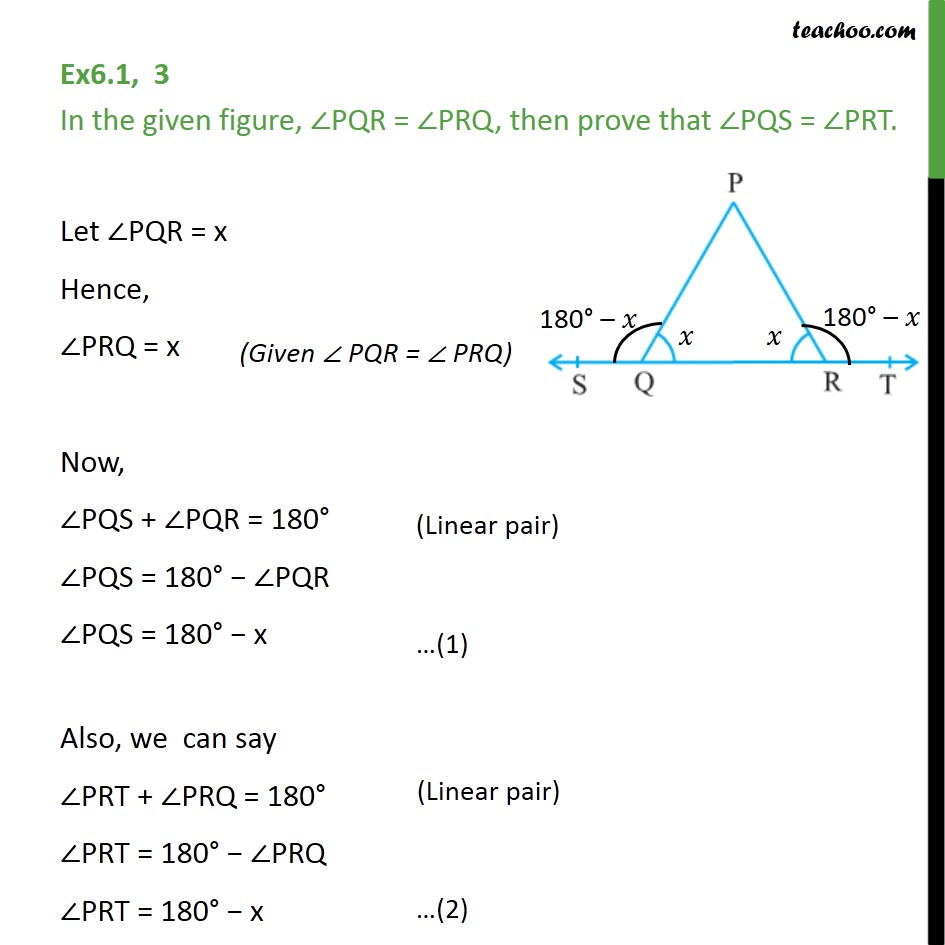Ex 6.1

Chapter 6 Class 9 Lines and Angles
Serial order wiseLearn in your speed, with individual attention - Teachoo Maths 1-on-1 Class

### Transcript

Ex6.1, 3 In the given figure, ∠PQR = ∠PRQ, then prove that ∠PQS = ∠PRT. Let ∠PQR = x Hence, ∠PRQ = x Now, ∠PQS + ∠PQR = 180° ∠PQS = 180° − ∠PQR ∠PQS = 180° − x Also, we can say ∠PRT + ∠PRQ = 180° ∠PRT = 180° − ∠PRQ ∠PRT = 180° − x From (1) & (2) ∠PQS = ∠PRT Hence proved.